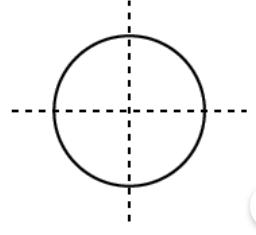Fill in the blanks:[] lines of symmetry can be drawn in the given 2D figure.

# Fill in the blanks:[] lines of symmetry can be drawn in the given 2D figure.Fill Out the Form for Expert Academic Guidance!l

+91

Live ClassesBooksTest SeriesSelf Learning

Verify OTP Code (required)

### Solution:

Concept: 2 lines of symmetry can be drawn in the given 2D figure. A line that passes through the center of a circle at any angle is said to be a line of symmetry. A Circle thus has an endless number of lines of symmetry. A line or lines of symmetry in various figures are not required to exist in every figure. Figures can occasionally have one, two, three, or four lines of symmetry. As a circle has infinite lines of symmetry, there are infinite lines of symmetry.
The Line of Symmetry is the line that divides a figure into two equally sized figures when it is drawn across its center. Along each of its diameters, the figure is symmetrical. the center can be traversed by an endless number of lines. If you give it any thought, this makes a lot of sense. Any circle's diameter, which divides the circle's circumference into two equal pieces, must pass through the center and two of its points. As a result, the circle has two lines of symmetry.
Hence, the answer is 2 lines of symmetry.

## Related content

 Area of Square Area of Isosceles Triangle Pythagoras Theorem Triangle Formula Perimeter of Triangle Formula Area Formulae Volume of Cone Formula Matrices and Determinants_mathematics Critical Points Solved Examples Type of relations_mathematics+91

Live ClassesBooksTest SeriesSelf Learning

Verify OTP Code (required)